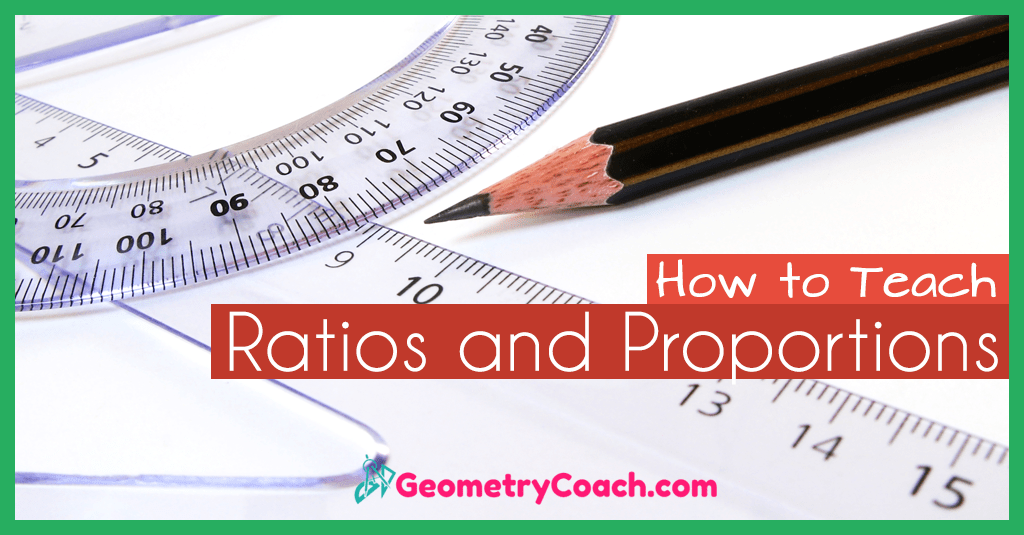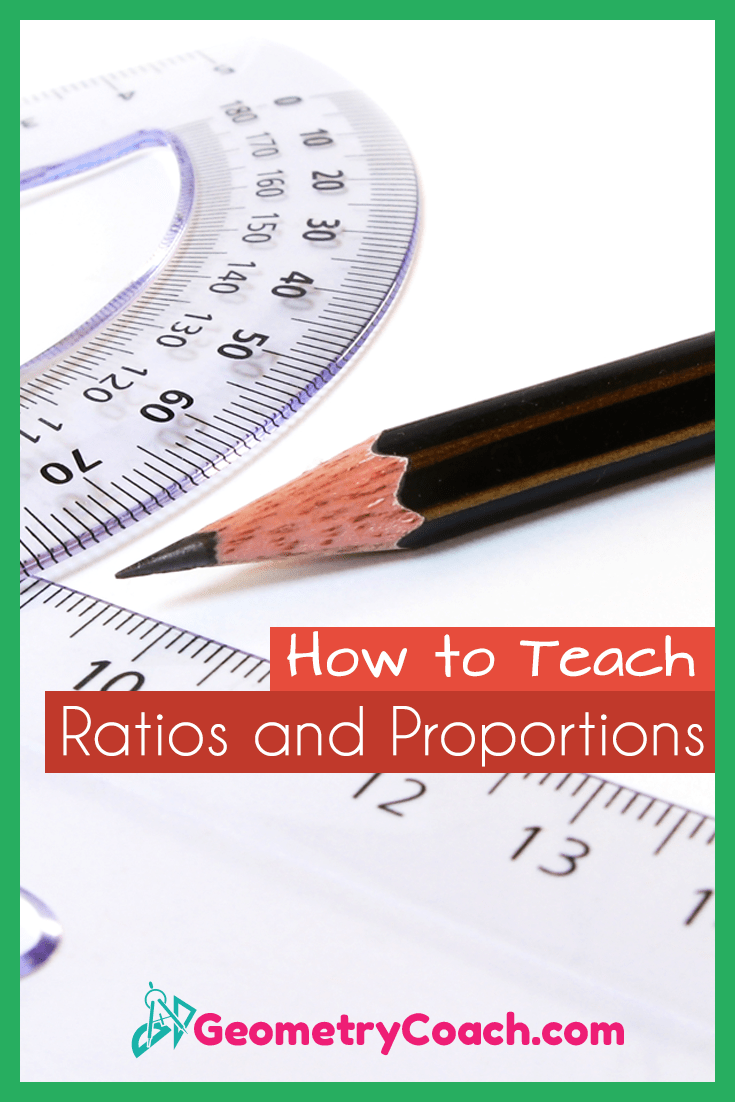# Ratios and Proportions – Bad Teacher!

This is a lesson where you’re going to have to do some corrective teaching. Odds are their previous teacher taught them “cross multiply and divide!”

This method of teaching ratios and proportions is no more than a trick! It shows nothing about knowing why the students are doing what they are doing. It is far more important to show them that the diagonals are equal instead. If they understand that the diagonals must be equal rather than cross multiplying and divide then they will be able to solve any proportion as they come along throughout different math classes.

## Do Not Use Tricks When Teaching Ratios and Proportions

What do I mean? “Cross multiply and divide” works if you are only using numbers. It does not work if the proportion contains multiple polynomials with variables in multiple parts of the proportion. If you were to cross multiply and divide something like that then you would also have to finish solving for the variable after you cross multiply and divide. Instead the steps to solving a Proportion should be

### Step 1:

Set the Product of the Diagonals Equal to Each Other

### Step 2:

Solve for the Variable

In conclusion, do all of your students future math teachers a favor and do not teach them to “cross multiply and divide”. Teach them to set the product of the diagonals equal to each other and then solve!

## Ratios and Proportions Worksheet, Word Docs,  & PowerPoints

To gain access to our editable content Join the Geometry Teacher Community!
Here you will find hundreds of lessons, a community of teachers for support, and materials that are always up to date with the latest standards.

## Ratios and Proportions Worksheet PDFs

7-1 Lesson Plan – Ratios and Proportions (Members Only)

7-1 Class Activity – Ratios and Proportions ( Members Only )

7-1 Online Activities – Ratios and Proportions (Members Only)

7-1 Video Lesson (Members Only)